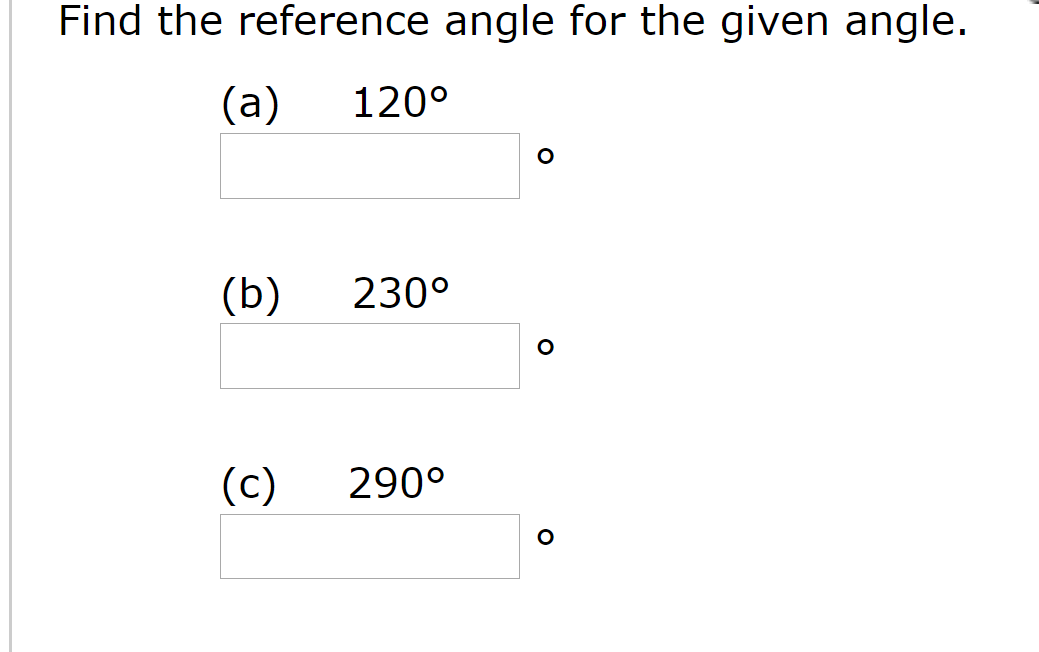# Find the reference angle for the given angle.(а)120°(b)230°(c)290°

Questionhelp_outlineImage TranscriptioncloseFind the reference angle for the given angle. (а) 120° (b) 230° (c) 290° fullscreen
check_circleExpert Solution
Step 1

Find the reference angle for the given angles.

Step 2

The reference angle is the angle that the given angle makes with the x-axis.

Part a: 120 degree.

Step 1: Determine the quadrant of given angle.

120 is lies between angle 90 and 180 (second quadrant)

So, terminal side is in quadrant 3rd.

Step 2 : Determine the operation.

Subtract the angle from 180 degrees.

Step 3

Part b: 230 degree.

Angle 230 is lies between 180 and 270.

So, terminal s...

### Want to see the full answer?

See Solution

#### Want to see this answer and more?

Solutions are written by subject experts who are available 24/7. Questions are typically answered within 1 hour*

See Solution
*Response times may vary by subject and question
Tagged in

### Other